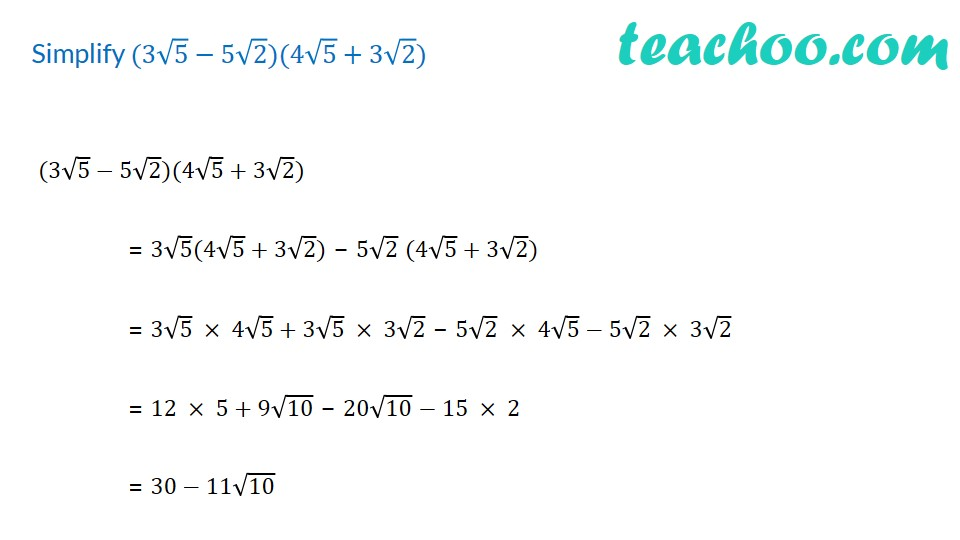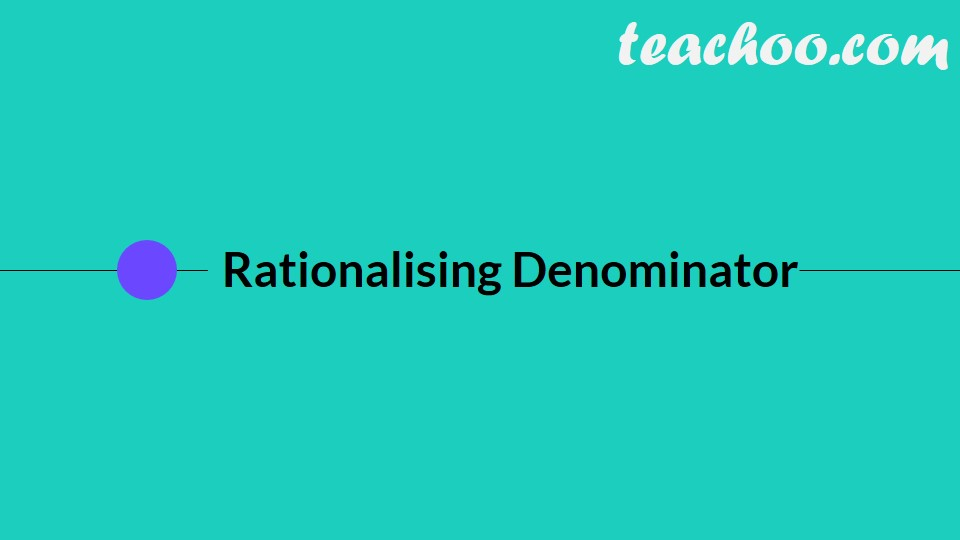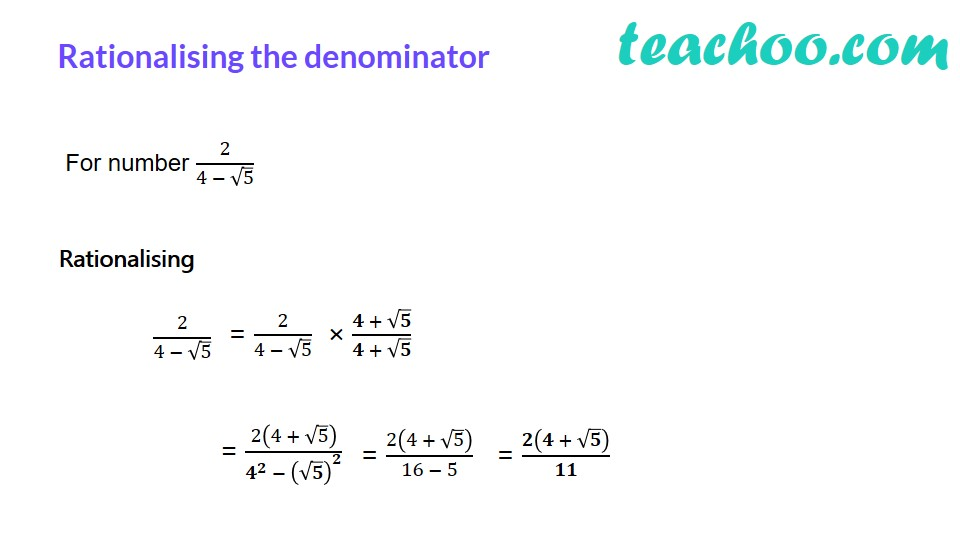1. Chapter 1 Class 9 Number Systems (Term 1)
2. Concept wise
3. Rationalising

Transcript

Simplify (3√5−5√2)(4√5+3√2) (3√5−5√2)(4√5+3√2) = 3√5(4√5+3√2) − 5√2 (4√5+3√2) = 3√5 × 4√5+3√5 × 3√2 − 5√2 × 4√5−5√2 × 3√2 = 12 × 5+9√10 − 20√10 − 15 × 2 = 30−11√10 Rationalising the denominator For number 2/(4 − √5) Rationalising 2/(4 − √5) = 2(4 + √5)/(𝟒^𝟐 − (√𝟓)^𝟐 ) = 2/(4 − √5) × (𝟒 + √𝟓)/(𝟒 + √𝟓) = 2(4 + √5)/(16 − 5)